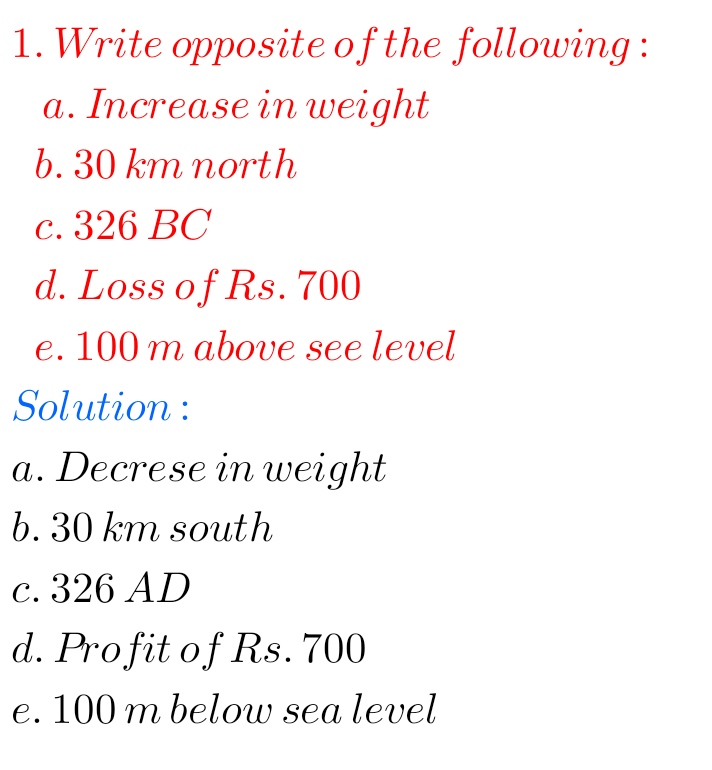## NCERT solutions for class 6th maths chapter 6/Integers/solutions for Integers, problems with solutions of Integers

NCERT SOLUTIONS FOR CLASS 6TH MATHS CHAPTER 6, INTEGERS NCERT class 6 maths chapter 6 Integers exercise 6.1 solutions are given. You should study the textbook lesson Integers very well. Observe the solutions and try them in your own method. You can also see. NCERT class 6 maths solutions Integers Exercise 6.1 Exercise 6.2 Exercise …